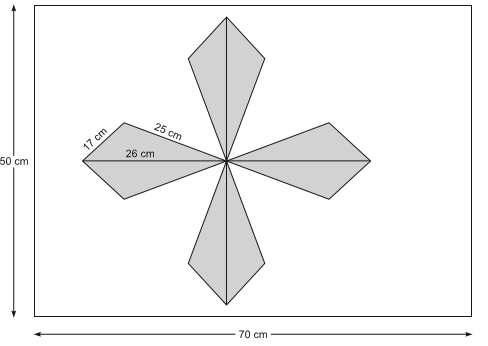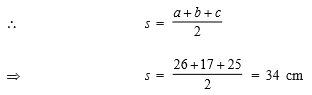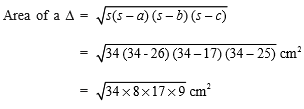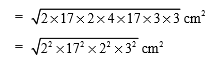Courses

# Value Based Questions- Heron’s Formula Class 9 Notes | EduRev

## Class 9 Mathematics by Full Circle

Created by: Full Circle

## Class 9 : Value Based Questions- Heron’s Formula Class 9 Notes | EduRev

The document Value Based Questions- Heron’s Formula Class 9 Notes | EduRev is a part of the Class 9 Course Class 9 Mathematics by Full Circle.
All you need of Class 9 at this link: Class 9

Question 1. Sarika participated in a drawing competition. She is required to make a design on a rectangular sheet of dimensions 50 cm × 70 cm. In the design she made 8 triangles, each of sides 26 cm, 17 cm and 25 cm as shown in the figure.(i) Find the total area of the design.
(ii) Find the remaining area of the sheet.
(iii) By drawing a design in a competition, which value is depicted by Sarika?
Solution.
(i) Sides of a triangle are: a = 26 cm, b = 17 cm and c = 25 cmUsing, Hero’s formula,= 2 × 17 × 2 × 3 cm= 204 cm2
∵ The design is having 8 equal (congruent) triangles.
∴ Total area of the design = 8 × (Area of one triangle) = 8 × 204 cm2
= 1632 cm2

(ii) Total area of the sheet =70 cm × 50 cm = 3500 cm2
∴ Remaining area of the sheet = (3500 – 1632) cm2
= 1868 cm2
(iii) Creativity.

,

,

,

,

,

,

,

,

,

,

,

,

,

,

,

,

,

,

,

,

,

;